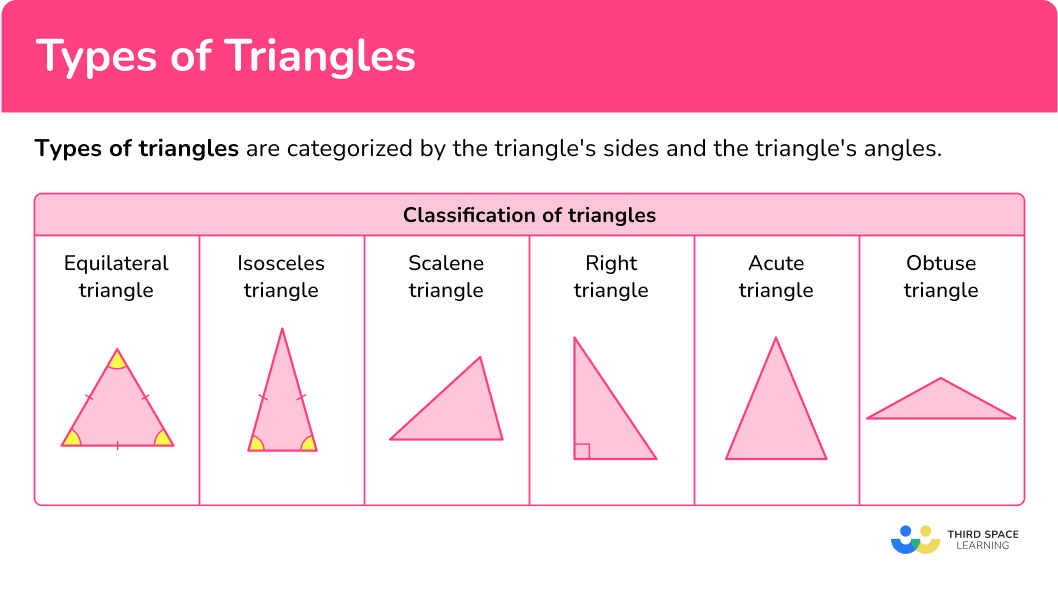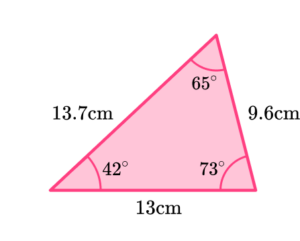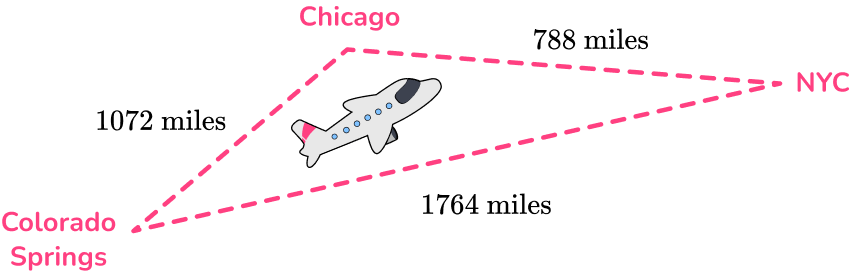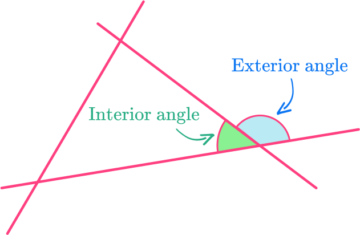Elementary math Geometry

Triangles

Types of triangles

# Types of triangles

Here you will learn about types of triangles, including their names, their properties, and applying them to problems.

Students first learn about triangles in kindergarten with their work in geometry, where they learn to reason with shapes and their attributes. They expand that knowledge as they move through elementary and middle school.

## What are types of triangles?

Types of triangles are categorized by the triangle’s sides and the triangle’s angles.

There are a lot of different types of triangles. Knowing the different classifications helps identify the type of triangle you have.

For example, classify the triangle by its sides and by its angles.

The triangle is marked with tick marks (dashes) on two sides and two angles. This means the triangle has two equal (congruent) sides and two equal (congruent) angles.

A triangle that has two equal sides and two equal angles is called an isosceles triangle.

The third angle is an angle that appears to be greater than, 90^{\circ} which means it is an obtuse angle, which means the triangle is also an obtuse triangle.

So, the full classification of this triangle is an obtuse isosceles triangle.

### What are types of triangles?## Common Core State Standards

How does this apply to 4th grade math?

• Grade 4 – Geometry (4.G.A.2)
Classify two-dimensional figures based on the presence or absence of parallel or perpendicular lines, or the presence or absence of angles of a specified size. Recognize right triangles as a category, and identify right triangles.

## How to classify a triangle

In order to classify a triangle by its sides:

1. Determine if the sides are congruent.
2. State the classification of the triangle.

In order to classify a triangle by its angles:

1. Determine the types of angles in the triangle and if they are congruent.
2. State the classification of the triangle.

In order to classify a triangle by its sides and angles:

1. Determine if the sides are congruent.
2. Determine the types of angles in the triangle and if they are congruent.
3. State the classification of the triangle.

## Types of triangles examples

### Example 1: classify a triangle by sides

Classify the triangle as scalene, isosceles, or equilateral.

1. Determine if the sides are congruent.

There are not any sides equal in measure, so there are no sides that are congruent.

2State the classification of the triangle.

A triangle with no sides that are equal is a scalene triangle.

(Note: This is also a right triangle. This can be shown using Pythagoras’ Theorem which you will learn in 8th grade.)

### Example 2: classify a triangle by sides

Classify the triangle as scalene, isosceles, or equilateral.

The triangle has two sides marked as equal, which means there are 2 congruent sides.

Since the triangle has two congruent sides, it is an isosceles triangle.

(Note: This is also a right triangle because there is a 90^\circ angle. It can be called a right isosceles triangle.)

### Example 3: classify a triangle by angles

Classify the triangle as acute, obtuse, or right.

Each of the angle measurements is different.

53^{\circ} is an acute angle.

55^{\circ} is an acute angle.

72^{\circ} is an acute angle.

Since all three angles are acute angles, the triangle is an acute triangle.

### Example 4: classify a triangle by angles

Classify the triangle as acute, obtuse, or right.

The triangle has one right angle.

Since the triangle has one right angle, it is a right triangle.

### Example 5: classify a triangle by sides and angles

The triangle has two marked congruent sides.

The triangle has one marked right angle and two marked congruent angles.

Since the triangle has two congruent sides and two congruent angles, it is an isosceles triangle.

Since the triangle has one right angle, it is a right triangle.

So, the triangle is a right isosceles triangle.

### Example 6: classify a triangle by sides and angles

Jill draws a triangle with three equal sides and three equal angles, like the one below. Classify the triangle by its sides and angles.

The sides of the triangle are congruent because Jill drew a triangle with 3 equal sides and marked them as congruent. So the triangle is equilateral.

The angles of the triangle are all equal in measure because Jill marked them all as 60^{\circ}. Since a 60^{\circ} angle is an acute angle, the triangle is acute.

The triangle Jill drew is an equilateral triangle. All equilateral triangles are considered equiangular, and equilateral triangles are considered acute triangles because all three angles are acute angles.

### Teaching tips for types of triangles

• Use hands on manipulatives to help students gain understanding of the different types of triangles.

• Use different sized toothpicks as a way for students to build understanding of the classification of triangles.

• Although worksheets provide students the opportunity to practice skills, using activities that require students to use rulers and protractors to sketch the triangles will help them formulate an understanding of the triangle classifications.

• Infuse digital activities such as the free resource, desmos.

### Easy mistakes to make

• Angles in triangles
Make sure you know your angle properties. Getting these confused causes quite a few misconceptions.
Acute angles are greater than 0^{\circ} and less than 90^{\circ} .
Obtuse angles are greater than 90^{\circ} and less than 180^{\circ} .
Right angles are equal to 90^{\circ} .

• Incorrect assumptions for triangles
Assuming that an isosceles triangle is also equilateral is an incorrect assumption. Equilateral triangles are also considered isosceles triangles because they contain two equal sides.

### Practice types of triangles questions

1. Classify the following triangle by its sides.Isosceles triangleScalene triangleEquilateral triangleRight triangleThe triangle is marked showing two equal or congruent sides. Triangles with two congruent sides is an isosceles triangle.

2. Which triangle has no equal sides?

Isosceles triangleEquilateral triangleIsosceles obtuse triangleScalene triangleBy definition, scalene triangles are triangles that have no equal sides and no equal angles.

3. A triangle has angle measures of 27^{\circ}, \, 31^{\circ}, and 122^{\circ}. Classify the triangle.

Right triangleEquilateral triangleObtuse triangleAcute triangle127^{\circ} is an obtuse angle and a triangle with one obtuse angle is considered to be an obtuse triangle.

4. Classify the following triangle.Equilateral triangleAcute scalene triangleAcute isosceles triangleObtuse scalene triangleThe triangle does not have any side lengths that are equal in measure.

42^{\circ} is an acute angle.

65^{\circ} is an acute angle.

73^{\circ} is an acute angle.

The triangle has three acute angles.

So, the triangle is an acute scalene triangle.

5. Dani is a pilot for United Airlines. Her flight path is from New York City to Chicago to Colorado Springs and then back to New York City. Classify the triangle formed by Dani’s flight path.Isosceles triangleRight triangleAcute triangleScalene triangleThe flight path makes a triangle that has three different side length measurements. Triangles with unequal side measurements are scalene triangles.

6. Billy measures the side lengths of a triangle to be 20 \, cm, \, 20 \, cm, and 31 \, cm. He measures the angles to be 40^{\circ}, \, 40^{\circ}, 100^{\circ}. What is the classification of this triangle?

Obtuse isosceles triangleAcute isosceles triangleRight isosceles triangleEquilateral triangleThe triangle has two side measurements that are equal and two angle measurements that are equal, so it is isosceles.

100^{\circ} is an obtuse angle.

So the triangle has one angle that is also obtuse.

Therefore, the triangle is classified as an obtuse isosceles triangle.

## Types of triangles FAQs

Can a triangle have more than one obtuse angle?

No, triangles can only have 1 obtuse angle because the sum of the interior angles of a triangle equal 180^{\circ}. If there was more than 1 obtuse angle in the triangle, the sum would be greater than 180^{\circ}.

Is an acute scalene triangle the same as a scalene acute triangle?

Yes, they mean the same thing which is a triangle with three acute angles and sides having different lengths.

Can a triangle be classified as both acute and obtuse?

No, triangles can’t be classified as both acute and obtuse because the definition of an acute triangle is a triangle with 3 acute angles, and the definition of an obtuse triangle is one that has 1 obtuse angle.

What is the Pythagorean theorem?

The Pythagorean theorem is used to find missing side lengths of a right triangle, a^2+b^2=c^2. Where a and b are the side lengths and c is the hypotenuse of the right triangle. The hypotenuse is the longest side of a right triangle. You will learn about the Pythagorean theorem in 8th grade.

Do triangles have exterior angles?

Yes, triangles have interior and exterior angles. The exterior angle is supplementary with the interior angle.• Area
• Perimeter
• 3D shapes

## Still stuck?

At Third Space Learning, we specialize in helping teachers and school leaders to provide personalized math support for more of their students through high-quality, online one-on-one math tutoring delivered by subject experts.

Each week, our tutors support thousands of students who are at risk of not meeting their grade-level expectations, and help accelerate their progress and boost their confidence.